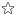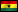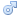quizzes  Forums# GoMathsToday-algebra

created by Innusah on 11 Oct 12, enabled by jimmyMedium level (73% of success)    30 questions - 56 players
Welcome To Go Maths Today1
What is between positive and negative?

2
What is - + -?

3
What is - + - + -?

4
What is - -?

5
What is + x -?

6
What is - x +?

7
What is - x -?

8
What is - x - x -?

9
What is - / -?

10
What is - / +?

11
What is + / -?

12
How many apples are there in each bag if you separate 37 apples into each of the 5 bags and there are 2 apples left?

13
What is -a^2 mean?

14
What is (-a)^2 mean?

15
What is equal to 2^-1?

16
Find the value of -2--2.

17
What is equal to 25%?

18
What is equal to -10+10?

19
Which of the following is the largest?

20
What is 0-1?

21
The product of a negative integer and negative 1 leaves the negative integer unchanged.

22
The product of two negative integers is always positive.

23
Kan and Dan have 77 cedis. If Dan has y cedis, what is Kan's share?

24
Dan is y years old now. What is his age three years ago?

25
What does "2 less than 4" mean?

26
Which of the following is an expresssion?

27
What does "greater than" mean?

28
In mathematics an "expression" does not carry a verb.

29
If the point A(x, y) is rotated 90 degrees counterclockwise, what is the image formed?

30
What is equal to a^2 - b^2?

Suggested byInnusahon Quizz.biz for https://sites.google.com/site/gmathstoday
Quiz.biz does not certify the quizzes to be reliable, contact Innusah !Comment this Quiz
If you want to rank and comment this quizz, login in or create an account !